Hi. I would like to know what is the square root of i , and i squared? I am looking for a response appropriate for secondary level students. Thank you, Wayne Hi Wayne The answer to your second question is straightforward since i is, by definition, one of the two numbers satisfying i squared is -1, the other is -i. Thus the answer to your second question is that i squared is -1. The first question requires more work but should be appropriate for secondary level students if they know some trigonometry. Complex numbers can be written in the form a+ib where a and b are real numbers. This gives rise to a graphical way to represent complex numbers in what is called the complex plane. Draw a coordinate system as you would for the Cartesian plane but label the horizontal axis the real axis and the vertical axis the imaginary axis. The complex number a+ib is then representated in the complex plane by the point with coordinates (a,b).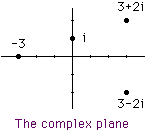For points on the unit circle (points at a distance 1 from 0) you can use some trigonometry to get a particularly useful representation. For a point z on the unit circle measure the angle (counterclockwise) between the positive real axis and the line segment joining z to 0. This angle, written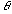, is called the argument of z and then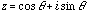. Now what happens when you multiply two such numbers? If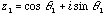and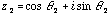then,expanding as you normally would, using the fact that i squared is -1 and using the double angle expressions for sine and cosine, gives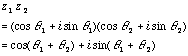Hence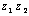is the complex number on the unit circle with argument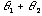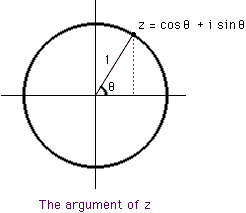Now back to your questions. First check, using this method, that i squared is -1. The complex number i has argument 90 degrees and hence i.i has argument 90 + 90 = 180 degrees. Hence i squared = cos(180 deg) + i sin(180 deg) = -1. For your first question you want z.z=i. Since the modulus of i is 90 degrees taking z with modulus 45 degrees will work, Hence z = cos(45 deg) + i sin(45 deg) and thus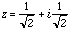There is, however, another possible choice for z, and that is with modulus 225 degrees, since doubling this angle also brings you around to i. Thus a second solution to i squared = -1 is then w=cos(225 deg) + i sin(225 deg) and thus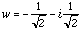You can check both solutions by calculating z times z and w times w. Cheers, Harley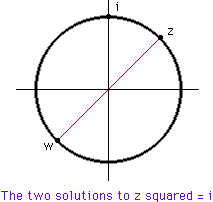Go to Math Central